﻿ Directional Flow Control with Multi-Electrode System Microplasma Actuator

# Journal of Biomedical Systems & Emerging TechnologiesOpen Access

• Research Article
• J Biomed Syst Emerg Technol 2017, Vol 4(2): 116

# Directional Flow Control with Multi-Electrode System Microplasma Actuator

Blajan M*, Ito A, Kristof J and Shimizu K
Shizuoka University, Nakaku, Johoku, Hamamatsu, 432-8561, Japan
*Corresponding Author: Blajan M, Shizuoka University, Nakaku, Johoku, Hamamatsu, 432-8561, Japan, Tel: +81-53-478-1443, Email: [email protected]

Received Date: Sep 22, 2017 / Accepted Date: Nov 20, 2017 / Published Date: Nov 27, 2017

### Abstract

The plasma actuator is a relatively new technology intended to replace mechanical actuators on aircraft or reduce drag. A small size dielectric barrier discharge microplasma actuator energized at low discharge voltage of 1.4 kV was applied for flow modification. Flow was measured using incense particles which were tracked by a highspeed camera. An AC voltage was applied to the multi-electrode microplasma actuator. The multi-electrode system allowed different electrodes to be driven independently, thus, due to the microplasma generation, leftward flow and upward flow were obtained by changing the configuration of the multi-electrode system. The high-speed camera measurements and particle tracking velocimetry (PTV) analysis showed the modification of the flow by microplasma at various time intervals. The experimental results were compared with the numerical simulations results obtained using our developed code considering the Suzen & Huang model. The numerical results were in agreement with the experimental results.

Keywords: Microplasma; Dielectric barrier discharge; Flow control; Actuator; Numerical simulation

#### Introduction

Plasma actuators have been investigated by many researchers in recent years and are one of the latest applications of nonthermal plasma. This type of actuator modifies the airflow due to the electrohydrodynamic (EHD) force. The EHD phenomenon occurs due to the momentum transfer from charged species accelerated by an electric field to neutral molecules by collision [1-5]. Although the technology is not yet implemented commercially there are advantages of plasma actuators over conventional actuators such as the lack of moving parts, simple construction and fast response due to fact that the EHD phenomenon is involved. The most common types of plasma actuator are dielectric barrier discharge (DBD) type and corona discharge type. By using a single DBD plasma actuator the induced flow could be up to 7 m/s and with multiple DBD plasma actuator design the value of induced flow could reach 11 m/s . Specific applications of flow control require also various types of plasma actuators. In the case of high-speed flow control, where an induced flow speed of more than 7 m/s is necessary, instead of using a single DBD plasma actuator the corona discharge could be used [6-10]. For applications such as turbulent boundary-layer control for skin-friction drag reduction millimeter size discharge gap DBD plasma actuators have to be energized at peak-to-peak voltages of about 7 kV [11-14]. Other studies regarding the applications of plasma actuators are turbulent boundary layer separation control, steady airfoil leadingedge separation control, oscillating airfoils dynamic stall control and circular cylinder wake control [15-20]. Various research papers are emphasizing that high values of the induced flow are desired. These high values conventionally are obtained by energizing the plasma actuators at tens of kilovolts which are difficult to insulate and also a large sized power supply is necessary. Also in order to have an effective actuation effect also a higher EHD force density is required. This could be achieved using micrometer order discharge gap plasma actuator that lowers the discharge voltage and consequently require a lower power. Separation flow control and drag reduction was achieved using microsized plasma actuators [21-23]. A microplasma actuator was developed in our laboratory . A similar electrode configuration was described by other authors but the required discharge voltages are more than 2 kV [25-28]. Microplasma is a type of dielectric barrier discharge nonthermal plasma that could be used as a replacement of conventional technologies for surface treatment of polymers, indoor air treatment, biomedical applications or flow control [29-33].

In microplasma actuators, because of the small dimensions, it is difficult to obtain experimental observations and measurements. Simulation could add valuable insights thus a simulation code was developed in our laboratory based on the Suzen & Huang [34-35] and Orlov  models, especially to investigate the flow near the electrode surface where due to the microplasma light emission the experimental measurements were difficult to carry out. The most common models for the numerical simulations of plasma actuator are the plasma fluid model and particle in cell model [37-46]. The Suzen-Huang model is a simplified phenomenological model which does not model the species transport equations but can replicate the effects of the actuator on the air . It is computational less expensive than solving the species transport equations and various researchers obtained results close to the experimental data [42-44,47]. Our first approach in modeling the microplasma actuator consisted in a simulation code based on Suzen- Huang model with less dense mesh and closed walls .

In this study, a multi-electrode plasma actuator was investigated which allowed the electrodes to be energized independently at various potentials or waveforms, thus a directional flow control was obtained. With the decrease in size the phenomenon is more difficult to observe and analyze. The novelty of the paper, consist in the experimental and numerical simulation study of a microplasma actuator energized only at 1.4 kV thus making this type of actuator more economical and suitable for easy and safe integration in various systems. The potential use for this microplasma actuator could be on the small drones. The microplasma actuator is thin and flexible and can be stick to any surface thus being ideal to place it in various parts of the exterior drone body where during various maneuvers in each area where the actuator was placed a different flow pattern could be necessary. The advantages of plasma actuator as a device with no moving parts are amplified by a greater flexibility in obtaining various flows by electronic switching. Moreover due to the small power supply and less required electrical insulation compared with macro plasma actuators there is less supplementary weight added to the drone. The findings of this paper are both experimental and numerical simulation results. First the experimental results show how the direction of the induced flow could be changed from leftward to upward flow using the microplasma actuator. In the second part the numerical simulation model and results are shown. The numerical simulation results have the purpose to validate the developed numerical simulation code after the comparison with experimental one and add valuable information of the phenomenon since due to the small size of the actuator and microplasma light emission the flow characteristics near the electrode were difficult to observe.

#### Experimental Setup

Due to the requirement of a low discharge voltage, the micro scale plasma actuator has the advantage of easy integration due to its small size. Figure 1 shows images and the schematic image of the DBD microplasma actuator .

Figure 1: Microplasma actuator. (a) Top view image with 20 strip-like electrodes; (b) Schematic cross-section view; (c) Image of the microplasma actuator discharge while electrodes HV2 and HV4 are energized; (d) Image of the microplasma actuator discharge while electrodes HV1 and HV4 are energized.

Alternating strip-like electrodes (topside electrode) are placed above a plate-like electrode (bottom-side electrode) having a 25 μmthick dielectric layer between them. The strip’s width was 200 μm and thickness was 18 μm. There were 20 strip-like electrodes and copper was used for the electrodes while polyimide film was used for the dielectric layer.

Due to the microscale gap, a high electric field (107~108 V/m) could be obtained at the topside electrode by application of a voltage of 1.4 kV. Such a low voltage is easy to insulate or control and contributes to the miniaturization of the system. Moreover, due to the use of the resin film, which is a lightweight and flexible polymer, the plasma actuator could be attached to surfaces of different shapes making it ideal for the flow control.

Flow was visualized by using the particle tracking velocimetry (PTV) . Approximately 0.3 μm tracer particles were used as incense smoke. The measurements were carried out in quiescent flow in a closed acrylic box. Nd YVO4 532 nm laser was utilized to visualize the flow. The incense smoke was used as tracer particle by other researchers and the Stoke number was calculated in order to determine the capability of the incense smoke to follow the flow . For our experimental condition and using 0.3 μm incense smoke tracer particles, the calculated Stokes number was of the order 10−5 for which the flow tracing accuracy has error less than 1% . The experimental setup is shown in Figure 2. The phenomena induced by the microplasma actuator were measured with a high-speed camera of 1280 × 128 pixel resolution. The high speed-camera captured a 25 × 4 mm2 area, and the recording frequency was set to 4000 Hz. The PTV results were obtained within the area of -5 mm ≤ x ≤ 5 mm and 0 mm ≤ y ≤ 2.0 mm with a resolution of 0.2 mm in the x and y-axes. For a PTV image with the velocity magnitude, two consecutive frames from the recorded movie of the flow were used as the data input in the software for image processing image and also the plugin for Particle Image Velocimetry .

The electrodes positioned in the left part of the actuator (x<0) are defined as HV1 and HV2 while the electrodes on the right part (x>0) were defined as HV3 and HV4, as shown in Figure 1. The electrodes that were covered by the dielectric layer were grounded.

High voltage power supply

The experiments were carried out by energizing electrodes HV2 and HV4 in order to obtain leftward flow and HV1 and HV4 in order to obtain upward flow, as shown in (Figure 1). In the case of leftward flow, electrodes HV1 and HV3 were at floating potential, and in the case of upward flow electrodes HV2 and HV3 were at floating potential. The applied sinusoidal AC voltage had an amplitude voltage of 1.4 kV and frequency of 20 kHz as shown in Figure 3.

The high voltage power supply was an inverter operated by 4 bilateral switches. The circuit’s outputs are perfectly synchronized due to the use of only one inverter. A LC resonance inverter was used as shown in Figure 4a. A micro controller is used to control the 4 bilateral switches thus 4 independent high voltages outputs are obtained as shown in Figure 4b. The schematic image of the bilateral switch is shown in Figure 4c. An FET switch (STW4N150; 1.5 kV breakdown voltage) and 4 diodes (RGP02-20; 2 kV breakdown voltage) were used.

Figure 4: High voltage power supply. (a) Schematic image of the LC resonance inverter; (b) Schematic image of the power supply; (c) Schematic image of the bilateral switch.

#### Results

The experiments were performed initially by energizing electrodes HV2 and HV4 up to 50 ms. After a steady state was achieved at 50 ms, the configuration of the energized electrodes was changed from HV2 and HV4 to HV1 and HV4.

Because of the small size of the microplasma actuator and the plasma light emission, the flow was difficult to observe and measure near the active electrodes, thus numerical simulations were carried out. Another factor influencing the measurements was the laser reflections from all HV electrodes.

Experimental results

Electrodes HV2 and HV4 were energized causing clockwise vortexes to appear near the active electrodes in the initial stages of the discharge at 2.5 ms, as shown in Figure 5a. The maximum flow velocity at this initial stage was approximately 0.4 m/s, but in the area near the energized electrodes, the measurements were not completely accurate due to the light emission of microplasma. Up to 10 ms the vortexes move to the left and up as number of turns in the vortex is increased. The same phenomenon was observed by Whalley & Choi  for a macro plasma actuator. After 10 ms, the vortexes start to join together and gradually developed in to a leftward flow.

(5a) Leftward flow. The active electrodes were HV2 and HV4. Clockwise vortexes appeared at 2.5 ms. The vortexes join together (10 ms) and finally leftward flow is established at 50 ms.
(5b) Upward flow. The active electrodes were HV1 and HV4. In the left part of the actuator the leftward flow is changed to counter-clockwise rotating vortexes (52.5 ms). After 60 ms the vortexes join together and form a bigger counter rotating vortex and at 100 ms the leftward flow generated in the right part of the actuator and rightward flow generated in the left part of the actuator collide in the centre resulting in upward flow.

Figure 5: PTV results. Up to 50 ms, leftward flow was obtained. After 50 ms the leftward flow gradually changed to upward flow.

The measured maximum velocity was 0.7 m/s. At 50 ms when a steady state was achieved, the leftward flow had widened towards the left side and the maximum velocity had reached 0.9 m/s. The PTV measurements were carried out up to a height of 2 mm where most of the phenomenon occurred. After 50 ms, the microplasma electrodes configuration was changed by switching from HV2 and HV4 as energized electrodes to a configuration where HV1 and HV4 were energized. At 52.5 ms, it could be observed that counterclockwise vortexes appeared above HV1 electrodes while above HV4 electrodes the leftward flow was maintained. A maximum flow velocity of 0.9 m/s was measured on the right, but due to the light emission from the microplasma, the measurements above electrodes HV1 were less accurate. At 60 ms, the vortexes above electrodes HV1 started to converge in one single vortex bigger in size rotating counter-clockwise. The maximum flow velocity was approximately 0.9 m/s. The result of the counter-clockwise vortex above electrodes HV1 and the leftward flow, above electrodes HV4 was upward flow, which appeared in the centre of microplasma actuator at 100 ms as shown in Figure 5b.

The magnitude of the flow of 0.9 m/s is not high compared with conventional type plasma actuators which are energized at tens of kilovolts. For airplanes are targeted conventional plasma actuators that are energized at tens of kilovolts and can generate flow up to 11 m/s, thus by scaling down, a flow of 0.9 m/s as we have obtained could be enough for small drones.

Simulation results

The measurements carried out in the area near the energized electrodes were not so accurate due to the light emission of the microplasma. Thus, the numerical simulations could give more information about the phenomenon near the active electrodes. Suzen & Huang model [34-35] was used for developing the simulation code. According to the model because of the atmospheric pressure microplasma and consequently weakly ionized gas particles, the potential V was considered in Suzen-Huang model as being composed from the potential ψ of the external electric field: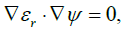(1)

and the potential φ potential of the net charge density ρc: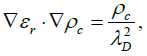(2)

where εr is relative permittivity and λD is Debye length.

Equations (1) and (2) are solved and thus we can calculate de body force: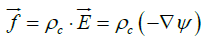(3)

Harmonic mean of the permittivity of the dielectric material which covers the grounded electrodes with εrd = 4 and air with εrair = 1 was calculated for the conservation of the electric field . For the potential ψ the outer boundary conditions were:(4)

For the potential φ the outer boundary conditions were: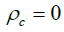(5)

As written in equation (2) we calculated the charge distribution over electrodes covered with dielectric material by considering these electrodes as source charge. In  the plasma distribution over the embedded electrode was prescribed by a distribution similar to a half-Gaussian distribution. In the case of more complex electrodes patterns this could be difficult to prescribe. If the embedded electrode is considered as a source for the charge density, solution of Eq. (2) gives the charge density distribution on the surface above the encapsulated grounded electrodes as measured in experiments and it is not required to give the charge density distribution on the surface and thus various shapes and orientations of electrodes can be easily modeled . The value of the source charge in our calculations was ρc =0.00751 C/m3. Also λD = 0.00017 m for the air, and λD = ∞ for the dielectric . After preliminary tests these values were chosen since it was a good agreement with the experimental data. The equation (3) was solved and body force Fx and Fy on x- and y-axes respectively was obtained. Furthermore Fx and Fy were introduced in the Navier-Stokes equations (6) and (7) in order to obtain the flow: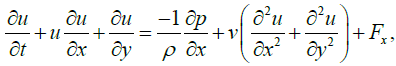(6)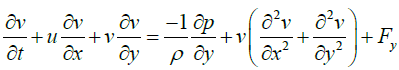(7)

The flow velocity has the components u and v on x- and y-axes respectively. In equations (6) and (7) ρ is the fluid density, p is the pressure and υ is the kinematic viscosity. We calculated the dynamic viscosity μ: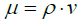(8)

Thus taking the value of air density ρ=1.177 kg/m3 and kinematic viscosity υ=1.57*10-5 m2/s, dynamic viscosity is μ=1.8*10-5 kg/ms.

The boundary conditions were considered open boundaries. The computational geometry is shown in Figure 6.

Figure 6: Computational geometry. HV2 and HV4 were energized in order to obtain leftward flow and HV1 and HV4 were energized in order to obtain upward flow. Encapsulated electrodes were at 0 V potential. (a) Schematic figure of the computational grid; (b) Schematic figure of the electrodes area.

The dimensions of the grid were 11.5 x 11.5 mm with 461 x 461 grid nodes. The grid spacing was chosen to be 25 μm in order to match the dielectric layer thickness. The simulation conditions were chosen to be close to the experimental one already shown in Figure 1. In the series of experiments shown in the previous chapter, the microplasma actuator had 20 line type exposed electrodes. Because simulating a large number of electrodes will increase the mesh dimensions and simulation time, in this study only eight exposed electrodes and four covered electrodes were considered. The numerical excitation conditions were the same as the experiments. The “push-push” theory was considered in the numerical simulations, thus the body force magnitude and direction obtained in the positive and negative half-cycle were very similar [15,36]. According to Orlov  the body force in the case of the discharge energized by a sinusoidal voltage the body force during one AC cycle will peak four times and the body force has always one direction from the exposed electrode to the encapsulated one. Therefore, for computational simplicity we considered the double rectified sine wave as the waveform of the body force, as shown in Figure 7.

Equations (1), (2) and (3) were solved to obtain the potential due to the external electric field ψ, the potential due to the net charge density φ, and the body force. The finite difference method was used for the discretization of the equations, which were computed before solving the Navier-Stokes equations. The maximum values of the external electric field, charge, and furthermore body force are shown in Figure 8. The highest intensity of body force was obtained near the active exposed electrodes HV1, HV2, and HV4 above the grounded electrodes. Julia programming language was used to write the simulation program . We have initially developed our simulation code using Python especially because the ease of use. Although Python is useful programming language for the physics simulation its speed can be a problem for more demanding simulations. In these types of cases a way to increase the speed is rewriting some parts of the code in lower level programming languages like C, C++ or Fortran. Another new alternative was Julia programming language which was promising an easy to write code as in Python and fast as C. Our simulation code written in Python was rewritten with relative ease in Julia and with some minor modifications we have obtained an increase in speed of about 10 times.

Figure 8: Electric potential, charge density and body force: Exposed electrodes HV=1400V; Covered electrode=0 V. Higher values of body force were obtained near the active electrodes HV. Potential Leftward Flow shows the potential ψ for the setup with active electrodes HV2 and HV4. Potential Upward Flow shows the potential ψ for the setup with active electrodes HV1 and HV4. Charge Density shows the charge density ρc for all setups. Body Force Leftward Flow shows the body force f for the setup with active electrodes HV2 and HV4. Body Force Upward Flow shows the body force f for the setup with active electrodes HV1 and HV4.

The Navier-Stokes equations were solved using the projection method in primitive variables on a collocated mesh. The projection method does not make use of the explicit equation for pressure. A Poisson equation for pressure is obtained after splitting the discrete form of each of the equations (6) and (7) in 2 equations, one with advection and diffusion terms and the other with pressure term. Furthermore we take the divergence of pressure term in order to derive the equation for pressure. The time step was 2 μs. The simulation conditions were similar with the experimental ones: thus, up to 50 ms, the energized electrodes were HV2 and HV4, and after 50 ms, the energized electrodes were HV1 and HV4. In Figure 9, the simulation results are shown when the energized electrodes were HV2 and HV4. The initial stages of the phenomenon are shown at 2 ms. setup with active electrodes HV1 and HV4.

A similar phenomenon was also observed in the experimental results. The vortexes that appeared above the covered electrode had a clockwise direction. At time t=10 ms the vortexes moved up, but flattened after 16 ms creating a flow directed leftwards.

The results at 50 ms appear to be a steady state which was also observed in the experiments. The calculated flow speed was approximately 0.93 m/s in some regions above the grounded electrode and approximately 0.85 m/s in the left region where the leftward flow widened. This is in agreement with the experimental results, where the measured flow speed by the PTV method was approximately 0.9 m/s. The experimental results in Figure 4 are showing only a part of the 20 electrode system microplasma actuator. In order to carry out a comparison with the simulation results it was chosen the middle area of the actuator. The phenomenon observed for the entire actuator was similar with what was observed in Figure 9. The leftward flow established after 50 ms had similar pattern.

In Figure 10, the simulation results when the energized electrodes were HV1 and HV4 are shown. After 50 ms, the configuration of energized electrodes was changed, thus leftward flow above the HV1 electrodes changed to counter-clockwise vortexes.

After 60 ms, the vortexes unite and form a single bigger vortex as shown also in the experimental results. The vortex gradually moves upward, and an upward flow is also obtained in the centre region due to the flow from the HV4 electrodes. The calculated flow speed in the regions above the grounded electrodes was approximately 1.05 m/s, and approximately 0.9 m/s in the centre region. This is in agreement with the experimental results, where the measured flow speed using the PTV method was approximately 0.9 m/s above the electrodes and also in centre region. At a distance of approximately 0.2 mm near the active electrodes, the experimental results could not show correct values due to the light emission from the microplasma. The simulation results show that the flow speed above the grounded electrodes was about 1.05 m/s instead of 0.9 m/s as obtained in the case of experimental results therefore a valuable information about the phenomena was obtained. If we consider in the case of PTV results the centre region values of the flow as being the most accurate we can conclude that the level of agreement was high. One of the input parameters in our calculations was the value of source charge which we choose it to be ρc =0.00751 C/m3 after preliminary testing the output flow values. Further research will be carried out to study the influence of this parameter on the flow.

After achieving a steady state while energizing electrodes HV2 and HV4, the configuration of the microplasma actuator was changed to HV1 and HV4 as the electrodes energized, a longer time was required to achieve a steady state for the upward flow. Thus, as shown in Figure 10 the steady state for the upward flow was achieved at approximately 120 ms.

The phenomenon of multi-electrode system microplasma actuator showed similar data when experimental and simulation studies were carried out. Due to the flexibility of the electrode arrangement up to 50 ms leftward flow was obtained and after 50 ms the leftward flow was changed to upward flow.

#### Conclusion

Directional flow control was achieved using microplasma actuator. The flow could be changed in different directions by modifying the configuration which energizes different electrodes of the actuator.

The experimental results showed a steady state for leftward flow was obtained within 50 ms and a measured maximum velocity of 0.9 m/s above the electrodes. By further changing the flow from leftward to upward in another 50 ms also a maximum velocity of 0.9 m/s was measured above the electrodes and in the main column of upward flow.

The calculated flow speed for the leftward flow at 50 ms was 0.93 m/s near the active electrodes and and approximately 0.85 m/s in the left region where the leftward flow widened. For the upward flow after 120 ms in the regions above the grounded electrodes the flow velocity was approximately 1.05 m/s, and approximately 0.9 m/s in the main column of upward flow. The numerical simulation results show the same phenomenon as the experimental one: initially clockwise rotating vortexes formed above the electrodes are evolving with the lapse of time in to leftward flow up 50 ms and further after changing the electrodes configuration the apparition of counter clockwise rotating vortexes in the left part of actuator that collide with the flow from the right part of the actuator and finally evolve in upward flow. Thus the developed numerical simulation program could be a valuable tool in the analysis of plasma actuator.

#### Acknowledgments

The authors would like to thank Professor Hitoki Yoneda from the University of Electro-Communications, Tokyo, for the fruitful discussions.

#### References

Citation: Blajan M, Ito A, Kristof J, Shimizu K (2017) Directional Flow Control with Multi-Electrode System Microplasma Actuator. J Biomed Syst Emerg Technol 4: 116.

Copyright: © 2017 Blajan M, et al. This is an open-access article distributed under the terms of the Creative Commons Attribution License, which permits unrestricted use, distribution, and reproduction in any medium, provided the original author and source are credited.

Select your language of interest to view the total content in your interested language

##### Recommended Journals
Viewmore
###### Article Usage
• Total views: 1792
• [From(publication date): 0-2017 - Dec 07, 2019]
• Breakdown by view type
• HTML page views: 1699Can't read the image? click here to refresh